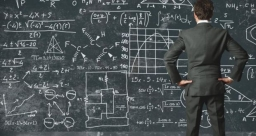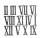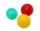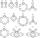# Two numbers

One number is by 79 larger than the other. If we divide the larger number by the smaller one, we get the ratio 5 and the remainder 11. Determine both numbers.

a1 =  96
b1 =  17
a2 =  113
b2 =  34
a3 =  147
b3 =  68

### Step-by-step explanation:Did you find an error or inaccuracy? Feel free to write us. Thank you!Tips to related online calculators
Do you have a system of equations and looking for calculator system of linear equations?
Do you solve Diofant problems and looking for a calculator of Diofant integer equations?

## Related math problems and questions:

• Two numbersThe difference between the two numbers is 74. If we divide a larger number by a smaller one, we get a quotient 7 and the rest of 2. Determine both numbers.
• Ratio of two unknown numbersTwo numbers are given. Their sum is 30. We calculate one-sixth of a larger number and add to both numbers. So we get new numbers whose ratio is 5:7. Which two numbers were given?
• Unknown number 5I think of an unknown number. If we enlarge it five times, then subtract 3, and the result decreases by 75%, we get one greater than the number. What number am I thinking of?
• Two-digit numberIn a two-digit number, the number of tens is three greater than the number of units. If we multiply the original number by a number written in the same digits but in reverse order, we get product 3 478. Find the original number.
• Digit sumThe digit sum of the two-digit number is nine. When we turn figures and multiply by the original two-digit number, we get the number 2430. What is the original two-digit number?
• The difference 2The difference between the two numbers is 25. The smaller number is 1/6th of the larger number. What is the value of the smaller number?
• The ratioThe ratio of two numbers is 4, and their sum is 75. Determine both numbers.
• Unknown integerFind the smallest integer: divided by 2, the remainder is 1. divided by 3, the remainder is 2. divided by 4, the remainder is 3. ... divided by eight, the remainder is 7, divided by 9 the remainder is 8.Divide number 135 into two additions so that one adds 30 more than 2/5 of the other add. Write the bigger one.
• ThereThere are two numbers on the screen - one in the blue and the other in the red box. In the beginning, both numbers are the same. With each beep, both numbers increase - by 1 in the blue field and by 3 in the red field. At one point, the number 49 appears
• The number 72The number 72 increase by 25%. By how much % will you have to reduce the number you created to get the number 72 again?
• Big numberThe hat is the remainder when dividing number 10 to 47 - 111 by number 9?
• DivisibilityDetermine the smallest integer which divided 11 gives remainder 4 when divided 15 gives remainder 10 and when divided by 19 gives remainder 16.
• The largerThe larger of two numbers is nine more than four times the smaller number. The sum of the two numbers is fifty-nine. Find the two numbers.
• Four integersFnd four consecutive integers so that the product of the first two is 70 times smaller than the product of the next two.
• RemainderA is an arbitrary integer that gives remainder 1 in the division with 6. B is an arbitrary integer that gives remainder 2 the division by. What makes remainder in division by 3 product of numbers A x B ?
• Unknown number 10Number first increased by 30%, then by 1/5. What percentage we've increased the original number?